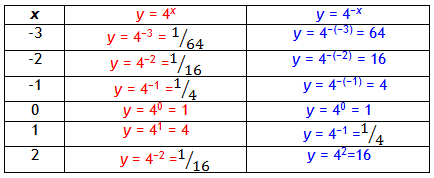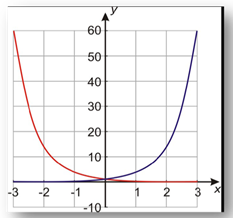Growth vs. Decay

An exponential growth graph starts very small and increases very quickly and the values end up very, very large. While an exponential decay graph starts with very large values, it decreases very quickly becoming very, very small values.

Example Graph the functions y = 4x and y = 4?x on the same coordinate axes.

Step 1. Make a table of values.*Note: The values of these two functions are reciprocals of each other.

Step 2. Graph the function by plotting points.*Note: The second function is a reflection of the first function across the y-axis. Recall from earlier discussions of transformations of graphs, that this is always true.